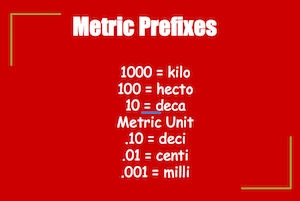# Metric Conversions: Lesson for Kids

Instructor: Jeremy Cook

I have been teaching elementary school for 16 years. I have extensive experience in lesson and curriculum development and educational technology.

Whenever we want to measure how long something is, how much it weighs, or how much liquid we have, we can use the metric system to do it. But what happens if we need to convert between larger and smaller units? This lesson will show you how.

## Metric Measure and Prefixes

When we want to measure how tall or wide or long something is, we are measuring length. In the metric system, each way we measure has a base unit that we start from. The base unit of length is the meter. A meter is about the length of a guitar.

For units larger than a meter, we multiply by 10, and to get smaller, we divide by 10. Each time we do this, we add a prefix to the word 'meter' to show the length. If we want a length smaller than a meter, we would cut it into 10 pieces. Those pieces would be called decimeters. 'Deci-' is the prefix for .10 of a meter.If we want to weigh something, or find its mass using the metric system, we would use the base unit called a gram. Just like with length, we either get larger by the power of 10 or smaller by 10. Each new unit simply adds a different prefix and the great thing is that the prefixes are the same. So what weighs a gram? If you hold two regular paperclips in your hand, you'll be holding a gram. If we want to weigh something larger, we would multiply a gram by 10, giving us 10 grams, or a dekagram (decagram).

Have you ever been to a baseball game? Each baseball stadium has a capacity, meaning it has a limit to the amount of people that can fill the space. When we measure capacity, we are usually talking about liquid. Have you been to a party and seen a big bottle of soda on the table? I'll bet you didn't know that the soda in that bottle is measured using the metric system. It's in liters. The base unit of capacity in the metric system is the liter. A liter is about the same as a quart of milk. And just like length and mass, we add the same prefixes to get larger or smaller units. So 100 liters would be a hectoliter.

To unlock this lesson you must be a Study.com Member.

### Register to view this lesson

Are you a student or a teacher?

#### See for yourself why 30 million people use Study.com

##### Become a Study.com member and start learning now.
Back
What teachers are saying about Study.com

### Earning College Credit

Did you know… We have over 200 college courses that prepare you to earn credit by exam that is accepted by over 1,500 colleges and universities. You can test out of the first two years of college and save thousands off your degree. Anyone can earn credit-by-exam regardless of age or education level.